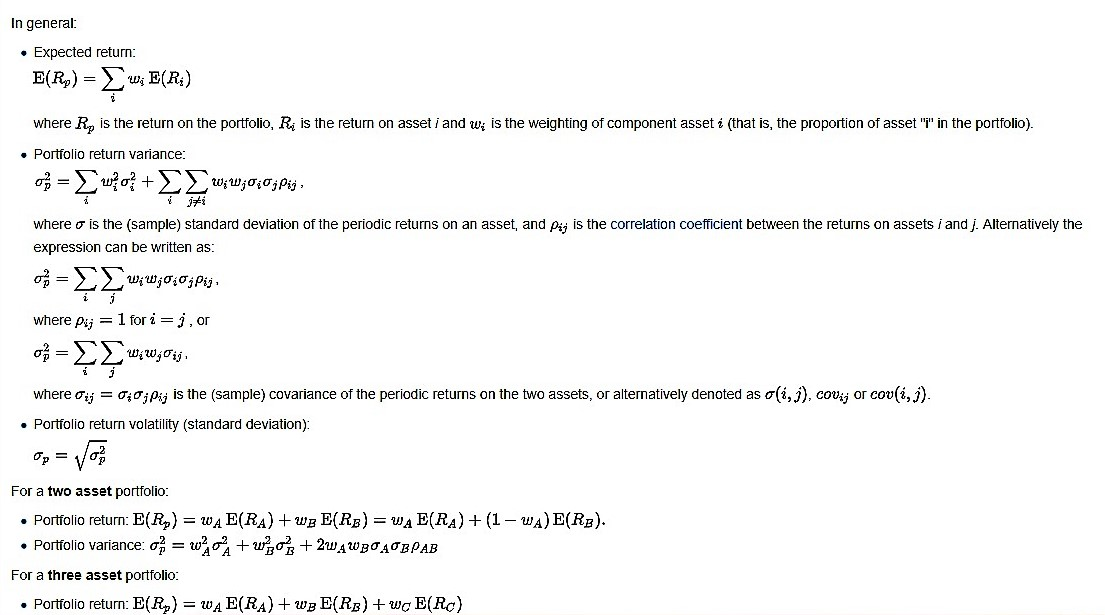# Portfolio Tools: Mean–Variance Analysis

Mean-variance analysis gives investors a framework to assess the tradeoff between risk and return as mean-variance analysis quantifies the relationship between expected return and portfolio variance (or standard deviation).

Modern portfolio theory (MPT), or mean-variance analysis, is a mathematical framework for assembling a portfolio of assets such that the expected return is maximized for a given level of risk. It is a formalization and extension of diversification in investing, the idea that owning different kinds of financial assets is less risky than owning only one type. Its key insight is that an asset’s risk and return should not be assessed by itself, but by how it contributes to a portfolio’s overall risk and return. It uses the variance of asset prices as a proxy for risk.

Economist Harry Markowitz introduced MPT in a 1952 essay, for which he was later awarded a Nobel Prize in Economics.

Risk and expected return

MPT assumes that investors are risk averse, meaning that given two portfolios that offer the same expected return, investors will prefer the less risky one. Thus, an investor will take on increased risk only if compensated by higher expected returns. Conversely, an investor who wants higher expected returns must accept more risk. The exact trade-off will be the same for all investors, but different investors will evaluate the trade-off differently based on individual risk aversion characteristics. The implication is that a rational investor will not invest in a portfolio if a second portfolio exists with a more favorable risk-expected return profile – i.e., if for that level of risk an alternative portfolio exists that has better expected returns.

This model is based on the following assumptions:

1. The return on an investment adequately summarizes the outcome of the investment.
2. The investors can visualize a probability distribution of rates of return.
3. The investors’ risk estimates are proportional to the variance of return they perceive for a security or portfolio.
4. Investors base their investment decisions on two criteria i.e., expected return and variance of return.
5. All investors are risk averse. For a given expected return he prefers to take minimum risk, obviously for a given level of risk the investor prefers to get maximum expected return.
6. Investors are assumed to be rational in so far as they would prefer greater returns to lesser ones given equal or smaller risk and risk averse. Risk aversion in this context means merely that, as between two investments with equal expected returns, the investment with the smaller risk would be preferred.
7. ‘Return’ could be any suitable measure of monetary inflows such as NPV, but yield has been the most commonly used measure of return, in this context, so that where the standard deviation of returns is referred to we shall mean the standard deviation of yield about its expected value.

Under the model:

Portfolio return is the proportion-weighted combination of the constituent assets’ returns.

Portfolio volatility is a function of the correlations ρij of the component assets, for all asset pairs (i, j).## One thought on “Portfolio Tools: Mean–Variance Analysis”

error: Content is protected !!